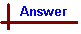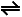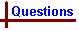.

 Questions - Second Law of Thermodynamics Your feedback on these self-help problems is appreciated. Click here to send an e-mail. Data table. Shortcut to QuestionsQ: 1 2 3 4 6 7 8 9 10 11 1 From the So298 tables, So298 for O2(g) = 205 J K-1 mol-1 while ΔHof for O2(g) = 0 kJ mol-1. Explain this difference.2 Inspection of the tables of standard entropy values of the elements at 298 K shows they are all +ve. Why is a negative value not found?3 Explain the trend shown in the following values of So which are listed for Cu(s): So273 = 31.0 So295 = 32.9 S0298 = 33.1, all in J K-1 mol-14 For liquid water at 298 K, So = 69.9 J K-1 mol-1 but for gaseous water at the same temperature, So = 188.7 J K-1 mol-1. Explain this difference.5 When oxygen gas dissolves in water, its entropy is reduced. So(O2(g)) = 205.0 J K-1 mol-1 while So(O2(aq)) = 110.9 J K-1 mol-1. Explain the reason for this. How would the entropy change for oxygen gas if it were mixed with some nitrogen gas?6 Using standard entropy tables, calculate ΔSo at 298 K for the spontaneous reaction Fe(s) + H2O(g) → Fe2O3(s) + H2(g)7 Calculate ΔSo at 298 K for the reaction H2(g) + 1/2O2(g) → H2O(g)8 Using entropy and enthalpy of formation tables, deduce whether the following reaction is spontaneous under standard conditions at 298 K: N2(g) + 3H2(g) → 2NH3(g)9 At 1 atmosphere, liquid and vapour phase water are in equilibrium at a temperature = 373 K represented by the equation H2O(l) → H2O(g) Show that ΔSouniverse = zero.10 Assuming standard conditions, use tables of free energy of formation to calculate ΔGo for the reaction H2(g) + 1/2O2(g) → H2O(g) at 298 K11 Calculate ΔGo for the conversion of graphite to diamond at 298 K and 1 atm pressure. The reaction is C(s)graphite → C(s)diamond12 A mixture of hydrogen, iodine and hydrogen iodide gases is placed in a closed container at 298 K. The initial pressures of the components are: P(H2) = P(I2) = 0.70 x 10-3 atm, P(HI) = 1.00 x 10 -3 atm. In which direction will the reaction proceed? The reaction equation is H2(g) + I2(g)2HI(g)13 The container is emptied and another mixture of the same gases at 298 K is introduced, the pressures being P(H2(g)) = P(I2(g)) = 0.50 x 10-3 atm and P(HI) = 15.00 x 10-3 atm. Deduce the direction of the reaction under these conditions.14 For the reaction I2(g) + Cl2(g) → 2ICl(g) at 298 K, calculate the thermodynamic equilibrium constant.15 Calculate the value of K for the reaction in Q 12 above.16 Assuming standard conditions, deduce the temperature at which the reaction N2(g) + 3H2(g) → 2NH3(g) becomes spontaneous.17 Calculate (i) DGo and (ii) K for the following reaction at standard conditions: 2Fe3+ + Sn2+ → Sn4+ + 2Fe2+Answers to Second Law Questions 1 The entropy change is that for oxygen gas at 0 K going to 298 K, a process which involves its molecules becoming more disordered due to their faster motion at the higher temperature. The enthalpy of formation of oxygen gas is, by definition, the enthalpy change for the process O2(g)(298 K)→ O2(g)(298 K) which is zero.2 As the temperature increases, the component atoms and molecules of the elements increase their motions and thus become more disordered - hence they have more entropy, so the sign of their entropies at higher temperature must be +ve.3 As the temperature increases, the atoms of copper move more rapidly and hence are more disordered. Consequently the entropy values increase as temperature increases.4 In the gas phase, the water molecules have much more freedom to move and are thus more disordered. Consequently the entropy value for liquid phase water is smaller than that for gas phase water at the same temperature.5 When a gas dissolves in water, its molecules associate with water molecules and this results in an increased degree of order for the system - hence the entropy value is smaller for the O2(aq). On the other hand, if two different gases are mixed and remain in the gas phase, they have less order as they are more randomly distributed than before they were mixed. Thus the entropy for oxygen in the gas mixture would be greater.6 ΔSo = ΣSoproducts - ΣSoreactants = [So(Fe2O3(s)) + So(H2(g))] - [So(Fe(s)) + So(H2O(g))] = [87.4 + 130.6] - [27.3 + 188.7] = 2.00 J K-1 mol-1 The +ve sign of the entropy agrees with the observed direction of spontaneity. It would have been impossible to be confident of correctly predicting the sign of ΔS for this reaction as there are 1 mole of a solid and 1 mole of a gas on both sides of the equation.7 ΔSo = ΣSoproducts - ΣSoreactants = [So(H2O(g))] - [So(H2(g)) + 1/2 So(O2(g))] = [188.7] - [130.6 + 1/2 x 205] = -44.4 J K-1 mol-1 The -ve sign of ΔSo indicates that the system becomes more ordered, which one might have expected given that two gases are becoming one gas in the reaction. This result shows that the sign of ΔSo for the reaction is not a totally reliable indicator of the direction of spontaneity either. The sign of the entropy change for a reaction does not in itself reliably indicate the direction of spontaneity, although it does accurately indicate the gain or loss of order in the system of reactants and products.8 To do this, need ΔHo, ΔSo for the reaction. (a) Calculate ΔHo. ΔHo = ΣΔfHo(products) - ΣΔfHo(reactants) = [2 x -45.9] - [0 + 0] = -91.8 kJ mol-1 = -91.8 x 103 J mol-1 (b) Calculate ΔSosystem ΔSosystem = ΣSo(products) - ΣΔSo(reactants) = [2 x 193] - [191.5 + 3 x 130.6] = -197 J K-1 mol-1 Note the -ve sign for this entropy change. This will require that ΔSsurroundings be +ve and larger in magnitude than 197 J K-1 mol-1. (c) The temperature of the system and surroundings must be taken as 298 K. Now, ΔSosurroundings = -ΔHo/T = -(-91.8 x 103)/298 = +308 J K-1 mol-1 Note that units for ΔH were converted to joules from kJ to match the entropy units. ΔSouniverse = ΔSosystem + ΔSosurroundings = -197 + 308 = +111 J K-1 mol-1 As this is positive, it shows that the reaction is spontaneous at 298 K, as is observed in practice.9 For the change L → R, ΔSosystem = 195.9 - 86.8 = 109.1 J K-1 mol-1 while for the surroundings, ΔSo = -Δ Ho/T = -440.7 x 103/373 = -109 J K-1 mol-1 [The Δ Ho value is the heat of vaporization of water at its boiling point] Thus Δ Souniverse = 109 + (-109) = 0 J K-1 mol-1 The signs show that for the forward process when equilibrium is established, the system has gained heat and becomes less ordered while the surroundings have lost exactly the same amount of heat and have become more ordered. For the reverse process, the sign of ΔSosystem would be -ve and the sign ofΔSosurroundings would be +ve, but again Δ Souniverse = 0. When a system is at equilibrium, neither direction of reaction is spontaneous, so there is no nett reaction taking place.10 ΔGo = ΣΔfGo(products) - SΔfGo(reactants) = (-228.6) - (0 + 0) = -228.6 kJ mol-1 The -ve sign shows that provided that the reaction is done at standard conditions, it would be spontaneous as ΔGo is -ve.11 Δ Go298 = S DfGo298 (products) - S DfGo298 (reactants) Therefore DGo298 = 2.900 - 0 = 2.900 kJ mol-1 Therefore the reaction is not spontaneous at standard conditions. Therefore the reverse reaction, conversion of diamonds to graphite is spontaneous at standard conditions, and DGo = -2.900 kJ mol-1. This illustrates the fact that a negative DGo for a reaction does not require the reaction to proceed at a measurable rate. The reaction may effectively be blocked by a high activation energy - just as well for all those with diamond rings.12 This question could be answered by comparing the value of Q which can be calculated from the data, with the value of the equilibrium constant. However, as no value for K was given, the alternative is to calculate the non-standard DG for the reaction under the given conditions and use the sign of DG to deduce the direction as follows. DG = DGo + RT ln Q Calculation of DGo: DGo = [2 x 1.7] - [0 + 19.36] = -15.96 kJ mol-1 Calculation of Q: Q = P(HI)2/(P(H2)P(I2)) = (1.00 x 10-3)2/[(0.70 x 10-3)(0.70 x 10-3)] = 2.04  Then DG = -15.96 x 103 + 8.314 x 298 ln (2.04) = -15.96 x 103 + 1.767 x 103 J mol-1 = -14193 J mol-1 or -14 kJ mol-1 The -ve sign shows the reaction at the specified conditions will proceed to the right to produce more HI. Note: (1) In the above calculation, the reaction quotient used was Qp because gases were involved. If the reaction involves species in solution, Qc would apply. (2) The value of R used was 8.314 because this has units of J K-1 mol-1 which are needed to be compatible with the units of DGo. (3) The conversion of kJ to J for the units of DGo to match those of R.13 Calculation of DGo: As in Q12, DGo = -15.96 kJ mol-1 Calculation of Q: Q = P(HI)2/( P(H2)P(I2)) = (15.00 x 10-3)2 /[ (0.50 x 10-3)(0.50 x 10-3)] = 900 Then DG = -15.96 x 103 + 8.314 x 298 x ln (900) = -15.96 x 103 + 16.85 x 103 J mol-1 = +0.89 x 103 J mol-1 or +0.89 kJ mol-1 The +ve sign shows the reaction under these conditions will proceed to the left, reversing the direction deduced under the conditions in Q12.14 DGo = [2 x -5.0] - [19.36 + 0] = -29.4 x 103 J mol-1 DGo = -RT ln K -29.4 x 103 = -8.314 x 298 x ln K ln K = 29.4 x 103/ (8.314 x 298) = 11.87 K = e+11.87 = 1.4 x 105 Such an extremely large K indicates the reaction is effectively complete.15 DGo = [2 x 1.7] - [ 0 - 19.36] = -15.96 kJ mol-1 = -RT ln K -15.96 x 103 = -8.314 x 298 x ln K ln K = 6.44 K = e+6.44 = 62816 Using the tables, DHo = -92.29 kJ mol-1, DSo = -198 J K-1 mol-1 From DHo = T DSo, T = - 92.29 x 103 /-198 = 466 K At T > 466 K, the magnitude of T DS would be bigger and would exceed the magnitude of DHo so DGo would be +ve, so the reaction was not spontaneous.17 (i) DGo = -nFEocell = - 2 x 96500 x (0.77 - 0.15) = -119.7 x 103 J mol-1 = -120 kJ mol-1 Note how a +ve Ecell will always result in a negative DG.   (ii) ln K = - DGo/RT = -(-119.7 x 103)/( 8.314 x 298) = +48.313 K = 9.6 x 1020 This very large value indicates that the reaction essentially goes to completion. However, the same method can be used to deduce K for reactions where it is small.